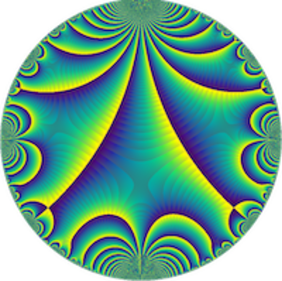# Uniform number theoretic estimates for fibers of polynomial maps over finite rings of the form Z/p^kZ — Yotam Hendel (Université de Lille)

Séminaire « Arithmétique »
M2 Kampé de Fériet

Let f:X \to Y be a morphism between smooth, geometrically irreducible Z-schemes of finite type.
We study the number of solutions #{x:f(x)=y mod p^k} for prime p, positive number k, and y \in Y(Z/p^kZ), and show that the geometry and singularities of the fibers of f determine the asymptotic behavior of this quantity as p, k and y vary.

In particular, we show that f:X \to Y is flat with fibers of rational singularities, a property abbreviated (FRS), if and only if #{x:f(x)=y mod p^k}/p^{k(\dim X -\dim Y)} is uniformly bounded in p, k and y. We then consider a natural family of singularity properties, which are variants of the (FRS) property, and provide for each member of this family a number theoretic characterization using the asymptotics of #{x:f(x)=y mod p^k}/p^{k(\dim X -\dim Y)}.

To prove our results, we use the theory of motivic integration (in the sense of uniform p-adic integration). If time allows, we will discuss these methods.

Based on a joint work with Raf Cluckers and Itay Glazer.